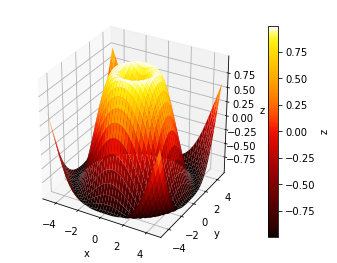# Surface 3d¶

Most examples work across multiple plotting backends, this example is also available for:

In :
import numpy as np
import holoviews as hv
hv.extension('matplotlib')## Define data¶

In :
# Make data.
X = np.arange(-5, 5, 0.25)
Y = np.arange(-5, 5, 0.25)
X, Y = np.meshgrid(X, Y)
R = np.sqrt(X**2 + Y**2)
Z = np.sin(R)

surface = hv.Surface(Z, bounds=(-5, -5, 5, 5))


## Plot¶

In :
surface.opts(colorbar=True)

Out: